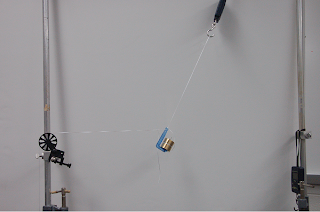Buy that special someone an AP Physics prep book, now with 180 five-minute quizzes aligned with the exam: 5 Steps to a 5 AP Physics 1

Visit Burrito Girl's handmade ceramics shop, The Muddy Rabbit: Mugs, vases, bowls, tea bowls... Fully revised November 2020 with items for the holiday season!

## 29 August 2011

### Equilibrium: quantitative demonstrations (or, how I teach vector math without teaching vector math)That's a 2 N weight hanging by two strings.  The left-hand rope passes over a pulley to a 1 N weight; the diagonal rope is attached to a digital scale at the top of the picture.

In Honors or AP Physics, I begin the year with equilibrium, not with motion.  In a few days, the class gets comfortable with free body diagrams, forces, and two-dimensional vector analysis.

Next comes motion in one dimension (graphs first, then algebra), followed by projectile motion.  Finally, we cover Newton's second law.  Since we did equlilibrium already, students are comfortable with free body diagrams and writing a vector sum of forces; since we did motion already, they already have some idea of what acceleration is.  Rather than a tough *new* topic, the second law becomes a way to review and solidify the first two topics.

The picture shows my third or fourth demonstration of the school year.  We begin by equating horizontal tensions in ropes pulling on a stationary block.  Next, I hang a 200 g mass vertically to find the tension in the supporting rope.  Easy stuff, so far.

And then, I attach a horizontal rope over a pulley attached to a 1 N weight. (In the picture, the hanging weight on the left is below the table, and out of the frame.)  When we make the free body diagram, everyone's comfortable with the weight of the 2 N weight acting down, and the horizontal rope pulling leftwards.  We draw the arrow representing the diagornal rope's force at an angle, of course.  Then what?

The class has already been taught that in equilibrium, up forces = down forces and left forces = right forces.  I ask, which is this diagonal rope, an up force or a left force?  Someone always comes up with a reasonable answer:  "both."  I redraw the free body diagram, with the tension in the diagonal rope replaced by two arrows, one up, and one to the side.

The class is totally comfortable with the upward component being equal to the mass's weight of 2 N; and with the leftward component being equal to the weight hanging over the pully of 1 N.  (They're also totally comfortable with me using the term "component" without preamble.)  It only takes a suggestive diagram to get someone to suggest that the resultant tension in the rope itself will be not 2 N + 1 N, but the pythagorean sum of 2.2 N.

The clincher comes when  I call a student to the front to read the digital scale attached to the rope.  It reads... 2.2 N.  Physics works.  I can even predict (and then measure) the angle made by the diagonal rope.

The key to this whole process is that I'm *not* just telling the class how to solve abstract vector addition problems.  I'm not telling them anything at all, really; I'm drawing diagrams and asking questions, getting someone in the class to suggest the next step wherever possible.  I model the correct problem solving method for equilibrium problems on the board, of course, but everything I do flows naturally from the fundamental principles of equilibrium: up = down, left = right.  I don't use any words like "vector" or "reference frame" or "coordinate system." The only technical term I'm introducing is "component," which was introduced organically.

The final demonstration with this setup involves adding a "mystery weight" to the stuff hanging over the pulley.  I measure the new angle that the diagonal rope makes, so I have a chance to suggest how to use sines and cosines to "break an angled force into components."  We predict the reading in the spring scale, and the amount of mystery weight that I added.  Once again, physics works.